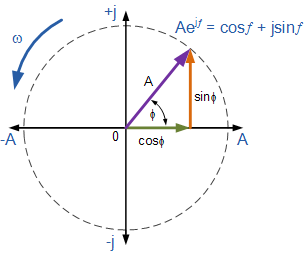# Roots of Unity (and Beyond)In this note, we will explain the mechanics for solving the roots of a complex number. It begins with a simple argument, then many elegant results follow.

We begin with the complex plane, where the vertical axis represents the imaginary numbers and the horizontal axis represents the real numbers (as pictured above). Normally, we define a complex number as $z = a+bi$, where $a, b$ are real numbers. We can also express the number in polar coordinates: $r = \sqrt{{a}^{2}+{b}^{2}}$ and $\theta = arctan\left( \frac{b}{a} \right).$

(Sorry, engineers like to use phi for angles, and j for imaginary numbers)

If we treat $a$ and $b$ as the components of a vector with magnitude $r$ from the origin, we can represent all imaginary numbers in polar form $z=r\left(cos \theta + isin \theta\right).$

By the well-known Taylor expansions of $cos x$, $sin x$, and ${e}^{x}$, we can easily show that ${e}^{i\theta} =\left(cos \theta + isin \theta\right).$

See proof

By treating this identity from a trigonometric perspective, we discover that ${e}^{i2\pi k} =1$; hence, if we solve for ${z}^{n} =1$, it follows that ${r}^{n}{e}^{in\theta} = {e}^{2\pi ik}.$ This implies that $r=1$ and $\theta = \frac{2\pi k}{n}$ where $k =0,1,2,...,n-1$. Consequently, the roots of unity are $cos\left(\frac{2\pi k}{n}\right)+isin\left(\frac{2\pi k}{n}\right).$

But what do we do to solve the roots of any complex number? Well, we have to make a transformation by finding the ${n}^{th}$ root of $r$ and adding $\frac{2\pi k}{n}$ to the angle value for the particular complex number! To visualize this, we look back at the diagram and notice the following:

1)For $z = 1$, the initial angle $\theta$ is zero. However, for $z=a+ib$ we have $\theta = arctan\left( \frac{b}{a} \right)$.

2)For $z = 1$, the magnitude is one. However, for $z=a+ib$ we have $r = \sqrt{{a}^{2}+{b}^{2}}$.

Hence the roots of a complex number are

${r}^{\frac{1}{n}}\left[cos\left(\theta +\frac{2\pi k}{n}\right)+isin\left(\theta + \frac{2\pi k}{n}\right)\right]$

for $k = 0,1,2,...,n-1$.

Check out my other notes at Proof, Disproof, and DerivationNote by Steven Zheng
6 years, 9 months ago

This discussion board is a place to discuss our Daily Challenges and the math and science related to those challenges. Explanations are more than just a solution — they should explain the steps and thinking strategies that you used to obtain the solution. Comments should further the discussion of math and science.

When posting on Brilliant:

• Use the emojis to react to an explanation, whether you're congratulating a job well done , or just really confused .
• Ask specific questions about the challenge or the steps in somebody's explanation. Well-posed questions can add a lot to the discussion, but posting "I don't understand!" doesn't help anyone.
• Try to contribute something new to the discussion, whether it is an extension, generalization or other idea related to the challenge.
• Stay on topic — we're all here to learn more about math and science, not to hear about your favorite get-rich-quick scheme or current world events.

MarkdownAppears as
*italics* or _italics_ italics
**bold** or __bold__ bold
- bulleted- list
• bulleted
• list
1. numbered2. list
1. numbered
2. list
Note: you must add a full line of space before and after lists for them to show up correctly
paragraph 1paragraph 2

paragraph 1

paragraph 2

[example link](https://brilliant.org)example link
> This is a quote
This is a quote
    # I indented these lines
# 4 spaces, and now they show
# up as a code block.

print "hello world"
# I indented these lines
# 4 spaces, and now they show
# up as a code block.

print "hello world"
MathAppears as
Remember to wrap math in $$ ... $$ or $ ... $ to ensure proper formatting.
2 \times 3 $2 \times 3$
2^{34} $2^{34}$
a_{i-1} $a_{i-1}$
\frac{2}{3} $\frac{2}{3}$
\sqrt{2} $\sqrt{2}$
\sum_{i=1}^3 $\sum_{i=1}^3$
\sin \theta $\sin \theta$
\boxed{123} $\boxed{123}$

## Comments

Sort by:

Top Newest

@Steven Zheng Can you add this to Roots of Unity - Definition or Applications? Thanks!

Staff - 6 years, 8 months ago

Log in to reply

Thanks. A good presentation.

- 6 years, 8 months ago

Log in to reply

×

Problem Loading...

Note Loading...

Set Loading...